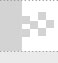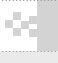Welcome, Guest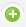Register |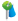Forgot Password |
 Karl Weierstrass “A mathematician who is not also a poet will never be a complete mathematician”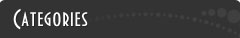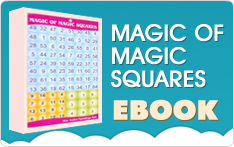How to solve the Magic Square PuzzleA typical 8x8 magic square obtained by using the mimic method consists of four 4x4 magic squares.

While the magic sum of an 8x8 magic square is 260. The magic sum of each 4x4 square is 130.In this, the 8x8 is a magic square and also each 4x4 is a magic square. It means that all rows, columns and the diagonals when added give the magic sum.

The magic sum of the 8x8 square will always be 260. The magic sum of the 4x4 will always be 130.

Also the center 2x2 of each 4x4 magic square will also give a total of 130. The centre 2x2 is marked in blue colour in the, given below.In addition to that each 2x2 as indicated below gives a total of 130 in this square.Cell :- Is a single box. It can contain numbers from 1 to 64.

Row: = Row is the horizontal group of cells. If you take the 8x8 as a whole then it consists of eight consecutive horizontal cells giving a sum of 260.

For a 4x4 square it consists of four horizontal cells giving a sum of 130.

Column: - It is the vertical group of eight cells. If you take the 8x8 as a whole then it consists of eight of eight consecutive vertical cells giving a sum of 260.

For a 4x4 square it consists of four vertical cells giving a sum of 130

Cage: - Is the group of cells marked by the perforated or dashed line. The sum of the group of cells marked should be equal to the number mentioned in the upper right hand corner of the cage.

Tips to solve the magic square puzzle

You can use these hints for solving this magic square puzzle.

All the 4x4 magic squares have one common property. This property was discovered by the great Mathematician Srinivasa Ramanujan. He applied this method to construct a Date Magic Square.NowFor example, the 8x8 magic square and each 4x4 square gives a magic sum of 130.

The four Numbers as highlighted in the following squares also give magic sum in any 4*4.That is
a + c + i + k = b + d + j + l = e + g + m + o = f + h + n + p = magic sum

Now we will take the first 4x4 square.Here all the rows, columns and the diagonals add up to 130.